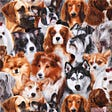# Context

Steel is one of the most important building materials of modern times. Steel buildings are resistant to natural and man-made wear which has made the material ubiquitous around the world. To help make production of steel more efficient, this competition will help identify defects.

Severstal is leading the charge in efficient steel mining and production. They believe the future of metallurgy requires development across the economic, ecological, and social aspects of the industry — and they take corporate responsibility seriously. The company recently created the country’s largest industrial data lake, with petabytes of data that were previously discarded. Severstal is now looking to machine learning to improve automation, increase efficiency, and maintain high quality in their production.

The production process of flat sheet steel is especially delicate. From heating and rolling, to drying and cutting, several machines touch flat steel by the time it’s ready to ship. Today, Severstal uses images from high frequency cameras to power a defect detection algorithm.

In this competition, you’ll help engineers improve the algorithm by localizing and classifying surface defects on a steel sheet.

If successful, you’ll help keep manufacturing standards for steel high and enable Severstal to continue their innovation, leading to a stronger, more efficient world all around us.

# Importing necessary libraries

`import pandas as pdimport numpy as npimport matplotlib.pyplot as pltimport seaborn as snsimport warningsimport matplotlib.pyplot as pltimport kerasfrom sklearn.model_selection import train_test_splitfrom tqdm import tqdmimport cv2from sklearn.preprocessing import OneHotEncoder,LabelEncoderfrom keras.utils import to_categoricalfrom keras.models import Sequentialimport tensorflow as tffrom keras.layers import Dense,Conv2D,Flatten,MaxPooling2D,Dropout`

`data = pd.read_csv("/kaggle/input/severstal-steel-defect-detection/train.csv")`

The dataset has three features ImageID , ClassID and EncodedPixels.

`data.shape(7095, 3)`

We have 7095 observations and 3 features.

# Countplot of ClassID

`data["ClassId"].value_counts().plot(kind = 'bar')data["ClassId"].value_counts()3    51501     8974     8012     247Name: ClassId, dtype: int64`

ClassID 3 has the maximum count.

# Image Augmentation

`l1=[]l2=[] for img,ClassId,EncodedPixels in tqdm(data.values):    image=cv2.imread("/kaggle/input/severstal-steel-defect-detection/train_images/{}".format(img),cv2.IMREAD_COLOR)    image=cv2.resize(image,(120,120))    l1.append(image)    l2.append(ClassId)`

Checking Random Steel images to check whether it is defected or not

`i = 15plt.imshow(l1[i])`
`i = 16plt.imshow(l1[i])`

Label encoding the input features and one hot encoding the target feature.

`encoder = LabelEncoder()X= np.array(l1)X = X/255y = encoder.fit_transform(l2)y = to_categorical(y)`

Splitting the data into 80% train set and 20% test set

`X_train,X_test,y_train,y_test=train_test_split(X,y,test_size=0.2,stratify=y,shuffle=True)print("x_train shape:",X_train.shape)print("x_test shape:",X_test.shape)print("y_train shape:",y_train.shape)print("y_test shape:",y_test.shape)x_train shape: (5676, 120, 120, 3)x_test shape: (1419, 120, 120, 3)y_train shape: (5676, 4)y_test shape: (1419, 4)`

# Building the CNN Model

`model=Sequential()model.add(Conv2D(32,(3,3),input_shape=(120,120,3),activation="relu"))model.add(MaxPooling2D(pool_size=(3,3)))model.add(Conv2D(64,(3,3),activation="relu"))model.add(MaxPooling2D(pool_size=(3,3)))model.add(Conv2D(64,(3,3),activation="relu"))model.add(MaxPooling2D(pool_size=(4,4)))model.add(Flatten())model.add(Dense(128,activation="relu"))model.add(Dropout(0.3))model.add(Dense(128,activation="relu"))model.add(Dropout(0.3))model.add(Dense(256,activation="relu"))model.add(Dense(4,activation="softmax"))`
`early_stopping = tf.keras.callbacks.EarlyStopping(patience=5,min_delta=0.001,restore_best_weights=True)`

# Compiling the model

`model.compile(loss=keras.losses.categorical_crossentropy,             optimizer=keras.optimizers.Adam(),             metrics=["accuracy"])`

# Fitting the model

`history = model.fit(X_train,y_train,epochs=15,validation_data=(X_test,y_test),batch_size=128,                    verbose=1, callbacks=[early_stopping])`

At 8th epoch we are getting an validation accuracy of 77% and validation loss of 0.65 due to callback(early stopping) it stopped at this epoch.

# Loss Curve

`history_df = pd.DataFrame(history.history)history_df.loc[:, ['loss', 'val_loss']].plot(title = 'Loss curve')`

# Accuracy Curve

`history_df = pd.DataFrame(history.history)history_df.loc[:, ['accuracy', 'val_accuracy']].plot(title = 'Accuracy curve')`

# Evaluating the Model

`result = model.evaluate(X_test, y_test)loss = resultaccuracy = resultprint(f"[+] Accuracy: {accuracy*100:.2f}%")45/45 [==============================] - 3s 74ms/step - loss: 0.5669 - accuracy: 0.7590[+] Accuracy: 75.90%`

# Result

We are getting an accuracy of 75.90% using CNN model to detect defective steel images. We can further improve our model using more complex layers. Considering it as a baseline model it further needs to be optimized to get a better performance.

# Hope you liked the analysis!

https://github.com/ratul442

# Kaggle Link of this project

https://www.kaggle.com/ratul6/steel-defect-detection-using-cnn

--

--

--

Software Engineer at Cyient | Data Science | Analytics | ML | AI | Deep Learning | NLP

Love podcasts or audiobooks? Learn on the go with our new app.

## 6 most commonly used Java Machine learning libraries## RTX 2080Ti Vs GTX 1080Ti: FastAI Mixed Precision training & comparisons on CIFAR-100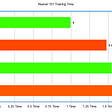## AI, Sustainability Tweets: Sentiment Analysis Using Pre-trained Models## How to Train SpaCy to Recognize Harry Potter Charms as Spell Entities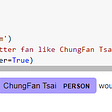## Potentiate Artificial Neural Networks: Thinking Learning (TL) and Smart Learning (SL) Theory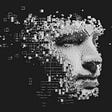## Reading the signs of migraines with ML: from algorithm to successful product development## Demystifying the GAN using a 1D Function (Keras)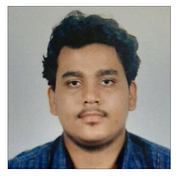## Ratul Ghosh

Software Engineer at Cyient | Data Science | Analytics | ML | AI | Deep Learning | NLP

## Human Activity Recognition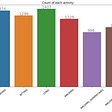## Dog Breed Classifier, Udacity Nano Degree Capstone Project